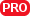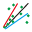#### File Exchange > Data Analysis >    Quantile RegressionAuthor:
OriginLab Technical Support
1/30/2019
Last Update:
3/21/2022
279
Total Ratings:
1
File Size:
268 KB
Average Rating:File Name:
QuantileReg.opx
File Version:
1.00
Minimum Versions:
Free
Summary:

Explore the relation between continuous or categorical independent variables and specified quantiles of a dependent variable.

Screen Shot and Video:
Description:

PURPOSE
This app can be used to identify the relation between independent variables and specified quantiles of a dependent variable. Independent variables include continuous or categorical independent variables. And the model can support interaction effects. It supports multiple bandwidth methods including bootstrap method. And for given predictors, it can predict the response from the built model.

INSTALLATION
Download QuantileReg.opx file, and then drag-and-drop onto the Origin workspace. An icon will appear in the Apps Gallery window.
NOTE: This tool requires OriginPro.

OPERATION

1. Make a worksheet for input data active. Click the Quantile Regression icon in the Apps Gallery window.
2. In the opened dialog, select a column from the worksheet as Dependent Variable in Input tab. Choose Continuous or Categorical Independent Variables. If you want to predict the response for test data, check Predict Response edit box, and select columns for Continuous or Categorical Independent Variables. Note that number of columns for Continuous or Categorical for test data must be same as that for input data.
3. In the Model tab, choose Main Effects or Custom Model from Model Type drop-down list. For the latter, you can custom interaction terms in the pop-up Custom Model dialog. Include Intercept option determines whether to include intercept parameter. If categorical independent variables are chosen, choose (1, 0) or (-1, 1) from Coding Type drop-down list.
4. In the Settings tab, type quantile values in Quantile(s) to Estimate (0-1) edit box. Quantile values must be between 0 and 1, and each quantile value must be separated by space. In Standard Error Settings group, choose Method to Estimate Covariance, four methods are available: IIDKernelHKS and Bootstrap XY. For first three methods, choose Bandwidth Method to determine the bandwidth: Hall-Sheather or Bofinger. For the last method, set an integer number to Bootstrap Replications. Specify Max Iter to define the maximum number of iterations.
5. In the Quantities tab, choose which quantities to compute. Quantities options include Standard ErrorConfidence Limitst-Value and Prob>|t| in Fit Parameters branch, Raw Sum of DeviationsMin Sum of Deviations and Pseudo R-Squared in Fit Statistics branch, Covariance MatrixFitted YResiduals and Predicted Y in Fitted Result branch. Confidence Level (%) in Fit Parameters branch must be between 0 and 100. Predicted Y in Fitted Result branch is available only Predict Response is checked in Input tab.
6. Click OK button, a report sheet and a report data sheet will be created.

ALGORITHM
The specified quantile of the dependent variable can be found by minimizing the sum of the loss function for residuals.
$$\min \displaystyle { \sum_{i} \rho_{\tau} \left( y_i-x_i^T \beta \right ) }$$
where $$\rho_{\tau}$$ is the piecewise loss function, and $$\beta$$ is the vector for fitted parameters.

$$\rho_{\tau}(z)= \begin {cases} (\tau-1)z, & \text{if } z<0 \\ \tau z, & \text{if } z \geqslant 0 \end {cases}$$.

If $$\tau = 0.5$$, the loss function becomes $$0.5 \displaystyle \sum_i \left \vert y_i-x_i^T \beta \right \vert$$ , and the regression becomes the method of least absolute deviations (LAD).

Preudo R-squared $$R_p^2$$ is similar to R-squared in the least squares method, but it subtracts quantile instead of mean, and uses the loss function instead of square of residuals:
$$R_p^2 = 1 - \frac \sum_i \rho_{\tau} \left( y_i - x_i^T \beta \right )}\sum_i \rho_{\tau} \left( y_i - Q(\tau) \right)$$ , where $$Q(\tau)$$ is the quantile of $$\tau$$ for input data $$y_i$$.

REFERENCE

Sample OPJU File
This app provides a sample OPJU file.  Right click on the Quantile Regression icon in the Apps Gallery window, and choose Show Samples Folder from the short-cut menu. A folder will open. Drag-and-drop the project file QRSample.opju from the folder onto Origin. The Notes window in the project shows detailed steps.
Note: If you wish to save the OPJU after changing, it is recommended that you save to a different folder location (e.g. User Files Folder).

NOTES
If there is only one continuous independent variable, fitted curve will be shown in the report.

 03/19/2022 BlancaR More clarification is needed regarding the methods used for calculation